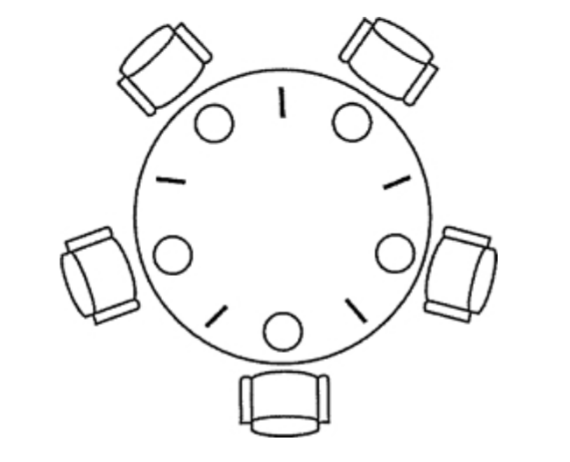# <操作系统>进程同步进程互斥

## 1.Intro

do {
entry section; //进入区
critical section; //临界区
exit section; //退出区
remainder section; //剩余区
} while (true)


## 3.实现临界区进程互斥的技术

### 3.1软件方法，即Peterson算法

// Px进程
flag[x]=1;  turn=y;
while(!flag[x] && turn!=x);
critical section;
flag[x]=0;
remainder section

//Py进程
flag[y]=1; turn=x;
while(!flag[y] && turn!=y);
critical section;
flag[x]=0;
remainder section;


### 3.2硬件方法，即硬件指令方法

TestAndSet指令：一个原子操作。功能是读出指定标志后把该标志设置为1.

bool TestAndSet(bool *lock)
{
bool old;
old  = *lock;
*lock = 1;
return old;
}


while TestAndSet (& 1 ock);
// 进程的临界区代码段;
lock=false;
// 进程的其他代码
Swap指令：该指令的功能是交换两个字节的内容。其功能描述如下。
Swap(boolean *a, boolean *b){
boolean temp;
Temp=*a;
*a = *b;
*b = temp;
}


## 4信号量机制

### 4.1信号量是什么

• 整型信号量
在设计操作系统的时候，我们会保留出两个原子语句操作：P(S)和V(S)，也可以称为wait(s)和signal(s)。wait(s)表示请求占用资源s，signal(s)表示回收资源s。这里使用s来表示某一空闲资源数量。
seaphore wait(s)
{
while(s<1);
s--;
}

seaphore signal()
{
s++;
}

• 记录型信号量
typedef struct {
int value;           //资源数目
struct process *L;   //整型变量
} semaphore;

void wait(semaphore S)
{
S.value--;
if(S.value>0)
{
add a process P to S.L;
S.value--;
}
}

void signal(semaphore S)
{
S.value++;
if(S.value<=0)
{
remove a process P from S.L;
wakeup(P);
}
}


### 4.2利用信号量实现进程互斥

semaphore S = 1;

// 进程A
P(S)
critical section
V(S)
// 进程B
P(S)
critical section
V(S)


### 4.3利用信号量实现进程同步

semaphore S = 0;

// 进程A
// …
x;  //语句x
V(S);

// 进程B
// …
P(S);
y;  //语句Y


## 5经典进程同步问题：生产者消费者问题

### 问题解决

semaphore vmutex = 1;
semaphore empty  = n;
semaphore full   = 0;

producer() {
P(empty);
P(vmutex);
critical section;
V(vmutex);
V(full);
}

customer() {
P(full);
P(vmutex);
critical section;
V(vmutex);
V(empty);
}


## 6经典进程同步问题：读者写者问题

### 问题解决

int       cnt   = 0;
semaphore mutex = 1;
semaphore rw    = 1;

writer() {
while (TRUE){
P(rw);
Writing;
V(rw) ;
}
}

while(TRUE){
P (mutex) ;
if (count==0)
P(rw);
cnt++;
V (mutex) ;
P (mutex) ;
cnt--;
if (count==0)
V(rw) ;
V (mutex) ;
}
}


## 7经典进程同步问题：哲学家进餐问题

### 问题陈述### 问题解决

#define N 5
semaphore mutex;
semaphore chopsticks[N];
memset(chopsticks,1,sizeof(chopsticks));

Pi() {
while(TRUE)
{
P(mutex);
P(chopsticks[i]);
P(chopsticks[(i+1)%N]);
eat;
V(mutex);
V(chopsticks[i]);
V(chopsticks[(i+1)%N]);
think;
}
}

posted @ 2020-09-06 11:42  dynmi  阅读(229)  评论(0编辑  收藏  举报